# How to Remove Duplicates From Array Using VBA in Excel?

• Last Updated : 09 Nov, 2021

Excel VBA code to remove duplicates from a given range of cells. In the below data set we have given a list of 15 numbers in “Column A” range A1:A15.  Need to remove duplicates and place unique numbers in column B.

Sample Data: Cells A1:A15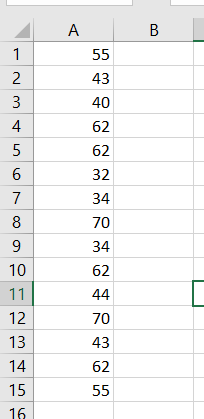Sample Data

Final Output: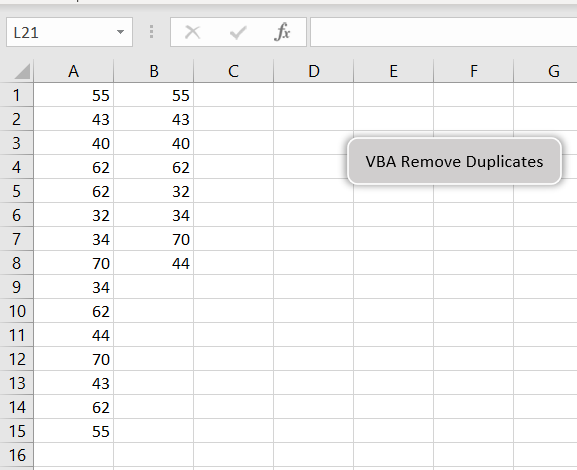VBA Code to remove duplicates and place into next column (B)

Declare Variables:

```'Variable Declarations
Dim nonDuplicate As Boolean, uNo As Integer, colA As Integer, colB As Integer```

Always first value will be unique, So A1 place to cell B1

```'Place first value to B1
Cells(1, 2).Value = Cells(1, 1).Value```

Initialize variables:

```'Initialize uNo = 1 since first number is already placed in column B; Assign True to the variable nonDuplicate

uNo = 1

nonDuplicate= True```

Since the first number is already placed in cell B1, Loop starts from A2 to A15.  Take each number from Column A and check with Column B (unique range)

```'Use for loop to check each number from A2 to A15
For colA = 2 To 15
For colB = 1 To uNo```

if the number is already placed in column B.  Assign False to the “nonDuplicate” variable.

```        If Cells(colA, 1).Value = Cells(colB, 2).Value Then
nonDuplicate= False
End If```

“nonDuplicate” is True then place to column B and increase uNo by 1

```    'if nonDuplicate is true, place cell value in column B and increase uNo = uNo + 1
If nonDuplicate = True Then
Cells(uNo + 1, 2).Value = Cells(colA, 1).Value
uNo = uNo + 1
End If```

Reset “nonDuplicate” variable

```'reset nonDuplicate to True
nonDuplicate = True```

Close for loop

`Next colA`

### Implementation:

Follow the below steps to remove duplicates using Excel VBA: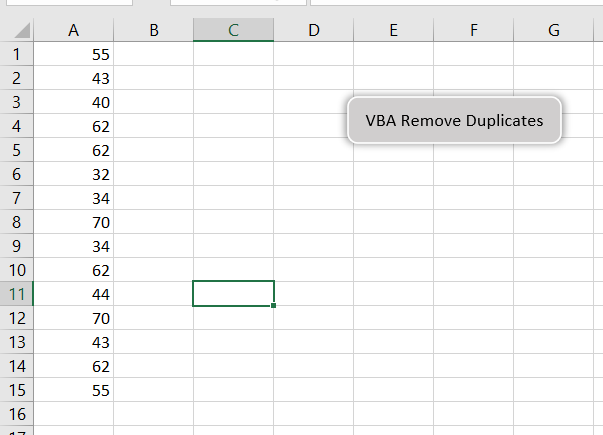Step 2: Right-click on “VBA Remove Duplicates” and “Assign Macro..”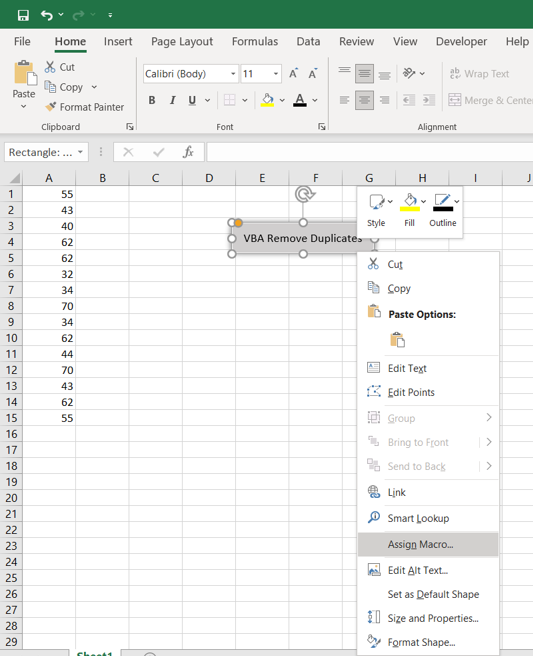Step 3: Select “removeDuplicates”, you can see a list of macros available in your workbook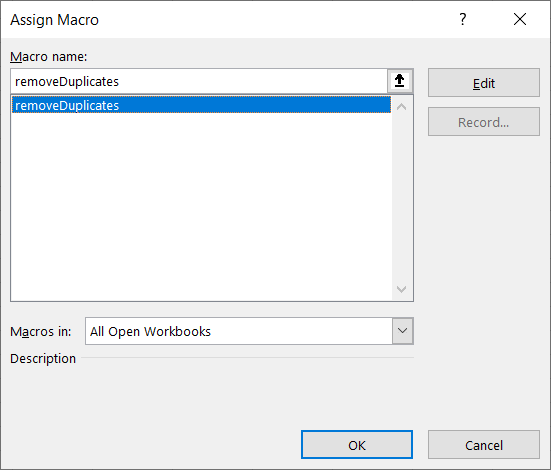Step 4: Save your excel file as “Excel Macro-Enabled Workbook” *.xlsm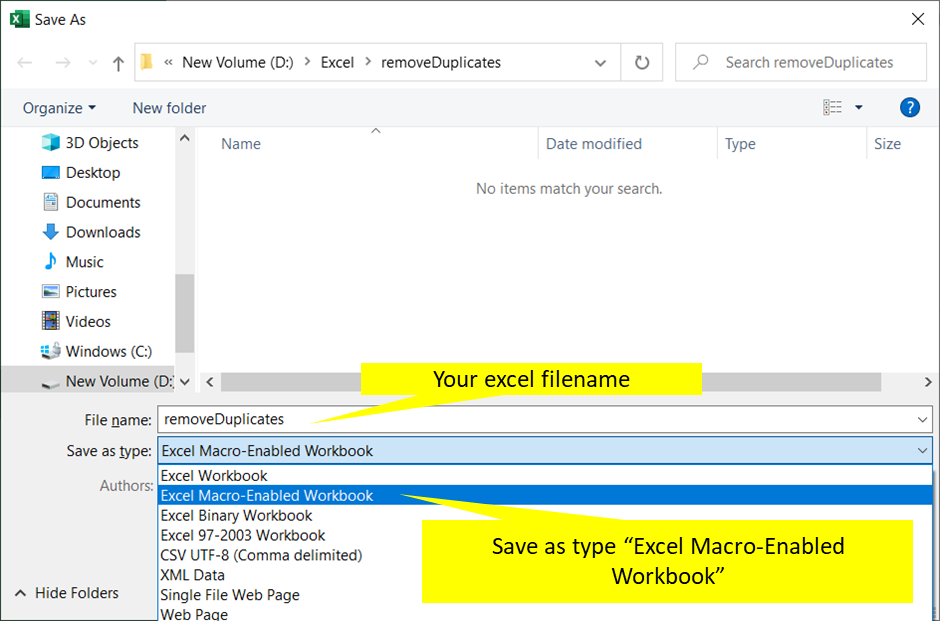Step 5: Click “VBA Remove Duplicates” to execute VBA code and see the output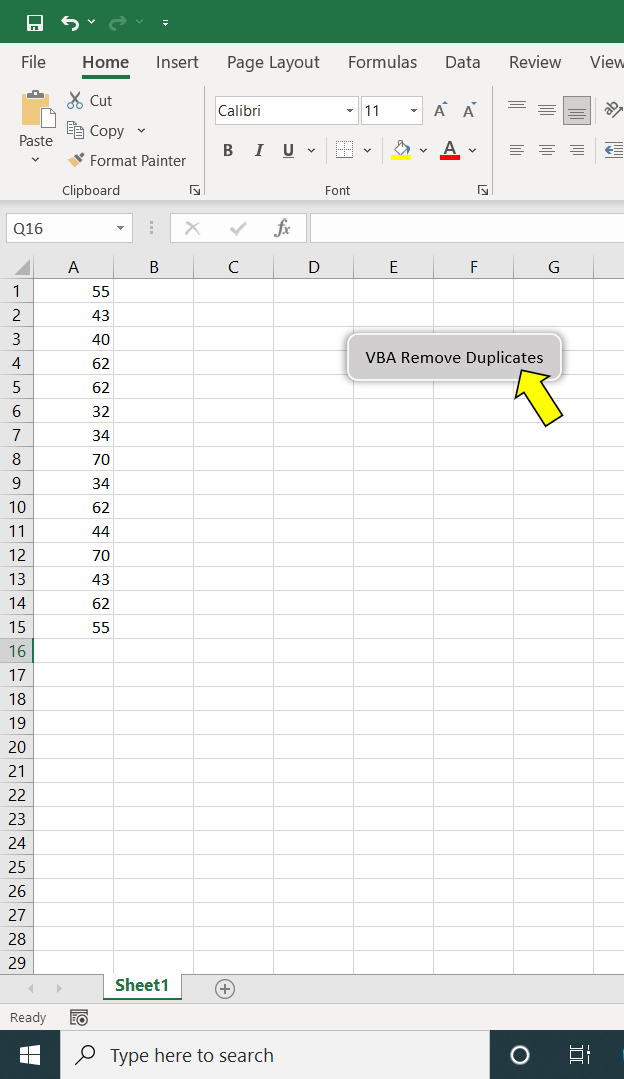My Personal Notes arrow_drop_up
Recommended Articles
Page :